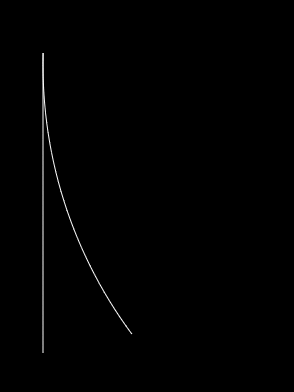# Intro to Visualizing Topology: Quotient

In topology we often think about what things look like. But fundamentally, these are mathematical objects and the jump to visualization is not trivial.

We often think in topology of gluing two different points together. This concept will become very useful. It means we are taking two points and considering them identical. We pretend there was only ever one point. We visualize this by only drawing one point.

We can glue together different points by using the “quotient”. Anything which has the same remainder under a quotient will be glued together. This idea will hopefully become clearer with examples.

Take the real line $$\mathbb{R}$$. What happens if we take the quotient of the real line with the integers? This is written $$\mathbb{R} / \mathbb{Z}$$, where $$\mathbb{Z}$$ represents the integers. This means we take every real number, and toss out the integer component. We glue together every point which has the same decimal expansion. So we glue $$3.2$$ to $$63.2$$ and $$\pi$$ to $$0.14159$$ and $$\pi +4$$, etc. The “remainder” of a real number with the integers is this decimal expansion. When you traverse the real number line, you go left and right by adding different numbers. If you can get from one number to another by adding an integer, then they have a special kind of equivalence; they have the same integer remainder.

How can we represent this object? Well, for each infinitely large “class” of real numbers which are glued together, we only draw one point. We can take a representative from each class and build our object like that. It should be pretty clear to see why the interval $$[0,1)$$ is representative of this object, since every real number will be glued to exactly one point on this interval. But why can’t we choose $$\pi$$ to represent the $$0.14159$$ class or $$63.2$$ to represent the $$0.2$$ class? Well, that’s because some of the structure of $$\mathbb{R}$$ is preserved. And when we analyze this structure, we observe something very special happens.

The basic structure of $$\mathbb{R}$$ is exactly what I touched on before: you traverse it by adding numbers together. Numbers which are close together should stay close together, regardless of how we quotient them. Say we start at $$1.6$$. If we want to get to $$1.7$$ we just add $$0.1$$ and if we want to get to $$3.7$$, we have to add $$2.1$$. But when we glue everything together, $$1.7$$ and $$3.7$$ will be represented by the same point, so the distance had better be the same! And this is what we find when we use $$[0,1)$$. $$1.6$$ gets glued to $$0.6$$ and $$1.7$$ and $$3.7$$ both get glued to $$0.7$$, and the distance is $$0.1$$ in both cases. Notice how $$2.1$$ and $$0.1$$ are glued together in this model, because they are separated by an integer.

Now, consider $$8.9$$ and $$9.1$$. You only need to add $$0.2$$ to $$8.9$$ to get $$9.1$$. But when we look at these two points on $$[0,1)$$, they appear much further. $$8.9$$ gets glued to $$0.9$$ and $$9.1$$ gets glued to $$0.1$$. Now to get from $$0.9$$ to $$0.1$$ we have to add $$-0.8$$. Ah! you say, $$-0.8$$ is glued to $$0.2$$ since they are an integer apart. Absolutely they are, but we see something important occurring. It turns out points towards the ends of $$[0,1)$$ are actually close together. So the quotient $$\mathbb{R} / \mathbb{Z}$$ is not, in fact, the line segment $$[0,1)$$. It is a circle! We must take the endpoints, $$0$$ and $$1$$ and glue them together because they are an integer apart!When you begin to visualize that, you see why we use the word gluing. The idea of plucking out points spread out across the number line and gluing them together might seem a bit bizarre, but it becomes much more concrete when you imagine the line segment folding around to make the endpoints meet into a circle.

As an exercise to the reader, try to imagine what shape we get when you take the entire Cartesian plane $$\mathbb{R}\times\mathbb{R}$$ and quotient out $$\mathbb{Z}\times\mathbb{Z}$$, meaning we ignore the integer component of every coordinate. How will you fold and glue your surface?